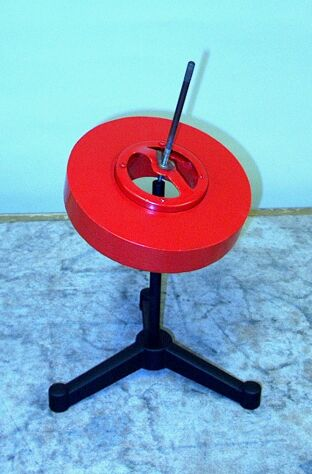A video of this demonstration is available at this link.

The shaft of this top is adjustable, so that you can set the pivot point at different heights relative to the center of mass of the top. You can set it below the center of mass, as for a normal top. If you set it above the center of mass, this reverses the direction in which the top precesses. If you set it at the center of mass, the top does not precess. To set the top spinning, you place it on the stand, and, pressing the shaft between your hands, move them in opposite directions.

The physics illustrated in this demonstration is similar or identical to that in demonstrations 28.54 -- Bicycle wheel as a top, 28.57 -- Bicycle wheel precession, and 28.63 -- Rotating wheel in suitcase.

When you set a top spinning, it has angular momentum, L = Iω, where I is the moment of inertia of the top, and ω is the angular velocity with which you have set it spinning. L points along the central axis of the top, and its sense is given by the right-hand rule. It points upward if the top is spinning counterclockwise as viewed from above, and downward if it is spinning clockwise as viewed from above.

A normal top spins on a point at its bottom, so that its center of mass is above that point. Unless the center of mass is exactly above the pivot point, gravity exerts a torque on the top, at the center of mass, about the pivot point. If the top is not spinning, it merely falls. When the top is spinning, however, it has angular momentum, and it cannot fall, but must go in the direction dictated by the change in angular momentum introduced by the torque exerted by gravity; it precesses.

If r is the position of the center of mass relative to the pivot point, the torque from gravity is τ = r × mg, where m is the mass of the top and g is the acceleration due to gravity. It points outward from the pivot point, along the plane on which the top sits, perpendular to r and mg, and rotates about the pivot point as the top precesses. The change in angular momentum equals this torque, or τ = dL/dt. Thus, the change in angular momentum – the precession – is in the direction of the torque exerted by gravity, and for a small time interval, ΔL = τΔt. L + ΔL has the same magnitude as L, but its head is displaced with respect to that of L by an angle φ, the angle made by the (horizontal) radii from L and L + ΔL to the vertical line above the pivot point.  (If we place the pivot point at the origin of a set of coordinate axes, this is the z-axis.) The rate at which L precesses, then, is ωp = Δφt. If we call the angle that L makes with the z-axis, θ, then the (horizontal) radius from the tip of L to the z-axis is L sin θ. (θ is also the angle that mg makes with r.) Since ΔL is very small compared to L (and to L sin θ), for an incremental change in φ, Δφ ≅ ΔL/L sin θ, and Δφ = τ Δt/L sin θ, so ωp = τ/L sin θ. τ = rmg sin θ, so ωp = rmg/L, or ωp = rmg/. (If we rewrite ωp = τ/L sin θ as τ = ωp L sin θ, we see that this torque is also the cross product of the precession velocity and the angular momentum: τ = ωp × L.)

As noted above, if you set the shaft of the Maxwell’s top so that the center of mass of the top is above the pivot point, it behaves as a normal top. If you give it a clockwise rotation (as viewed from above), it precesses in the clockwise direction. (To see this, you can imagine moving the tip of L in the direction of τ.) If you set it spinning counterclockwise (again, as viewed from above), it precesses in the counterclockwise direction.

If you set the pivot point below the center of mass of the top, this reverses the direction of the torque applied by gravity, and thus the direction in which the top precesses. Setting it spinning clockwise thus causes it to precess in the counterclockwise direction, and spinning it counterclockwise causes it to precess in the clockwise direction.

If you set the pivot point at the center of mass, gravity cannot exert any torque about the pivot point, because r now equals zero. There is thus no such torque, and however you set the top spinning, its axis remains fixed at whatever position you placed it when you released the top, and if you move it again, it remains in the new position. With the pivot set this way, the top also exhibits the interesting behavior that if you touch your hand against the shaft as the top is spinning, the shaft rolls along your fingers and follows the contour of your hand.

References:

1) Resnick, Robert and Halliday, David. Physics, Part One, Third Edition (New York: John Wiley and Sons, 1977), pp. 260-262.
2) http://hyperphysics.phy-astr.gsu.edu entry on precession of a spinning top.
3) http://hyperphysics.phy-astr.gsu.edu/hbase/rotv2.html#rvec4 entry on precession torque.